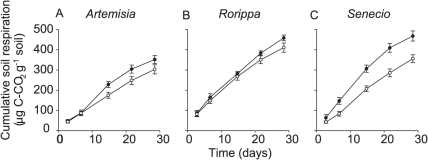# How to find standard deviation/error of average cumulative values?

I am a science student, however unfortunately a complete newbie in math and statistics. I am measuring soil respiration for my master thesis project and have a certain amount of carbon respired that I have measured over 30 day period. I plotted the respired carbon as a function of time in days and it looks similar to this:Each data point is a sum of the measured value at a certain day plus value of the previous measurement at a certain day (time). Now, I know how to calculate and report error bars for a basic data, but these are sums of values (cumulative values), so I am wondering how to estimate standard error or deviation for this type of data. How would I calculate and report error bars for my data in this situation?

P.S. This graph is just an example, not my actual data, but it is essentially the same.

$\text{Var}(X_1+X_2) = \text{Var}(X_1)+\text{Var}(X_2)+2\text{Cov}(X_1,X_2)$ and the $k-$variable case can be obtained recursively from that.
$f_n$ = $F_n$ - $F_{n-1}$
Where $f_n$ denotes the basic data and $F_n$ is the cumulative value